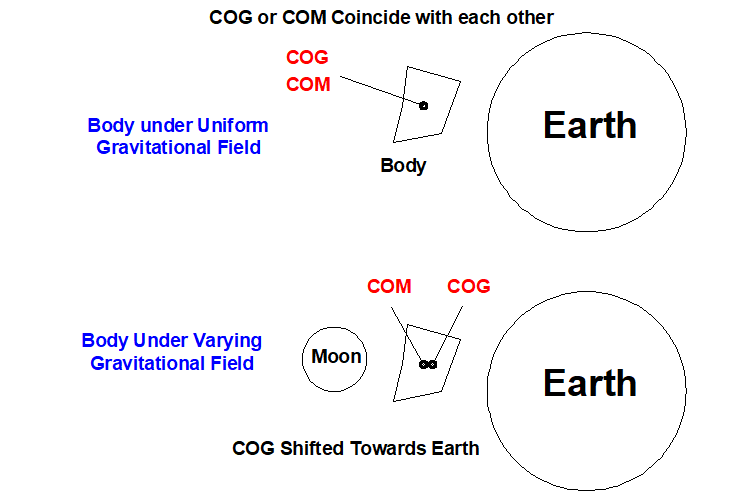# Difference Between Center of Gravity and Center of Mass

## What is Center of Gravity?

Center of gravity is defined as the point where whole weight of the body supposed to be acting irrespective of its position. For every position of a body we have only one center of gravity, it does not alter (changes) with the orientation of the body.

It is also defined as the point in a body about which the moments due to the force of gravity is zero.

It is the point through which the body can be

balanced perfectly against gravity. If we provide a support at this point against gravity than the body will come in equilibrium condition.

The center of gravity of a body is denoted by C.G. Or simply G. It may lie inside or outside of the body. For example if we have a solid cylinder than the G of the cylinder lie at the middle point of the cylinder, in this case the CG lies within the body. But if we have a hollow cylinder than in this case, the CG of the body lies in space i.e. outside the body. I think this examples has cleared your doubt how G of the body lies inside or outside of the body.

The COG of a body depends upon the value of ‘g’ (i.e. Gravitational Field) as the value of g changes the COG also changes. Lets take an example to understand it more clearly.Suppose we have  a body on earth and if we considered the force of gravity on the body is acting only due to earth. In that case we get a center of gravity on the body as shown in the diagram. But if we take the force of gravity of moon on the body too. Now the body is under two force of attraction and this time the field of gravity is not uniform across the body. This will result in the shift of the COG towards stronger field of gravity i.e. the COG of the body will shift towards the center of earth. But the COM remains unchanged due to the change in gravitation field.

## What is Center of Mass?

It is the point in the body where all the mass of the body is assumed to be concentrated. It is said to be the center of distribution of the mass in a body. The distribution of mass around this center of mass is uniform.

Center of mass of a body remains unchanged with the change in the force of gravity. It depends upon the quantity of the matter.

How Center of gravity and center of Mass coincide with each other?

When a body or object is present in uniform gravitation field than both the COG and COM coincide with each other.

## Difference Between Center of Gravity and Center of Mass in Tabular Form

 S.No Center of Gravity Center of Mass 1 The center of gravity is the point at which the whole weight of the body is supposed to be acting. The center of mass is the point at which the whole mass of the body is assumed to be concentrated. 2 A weight distribution of the body around COG is uniform. That is if we pass an axis through the COG, than the weight acting to the left is equal to the weight acting at right. The mass distribution around the COM is uniform. 3. The Center of gravity changes with the change in the force of gravity. It remains unchanged with the change in the gravitational field.

Here we have discussed about the difference between center of gravity and center of mass. If you enjoyed this information than don’t forget to like and share it.